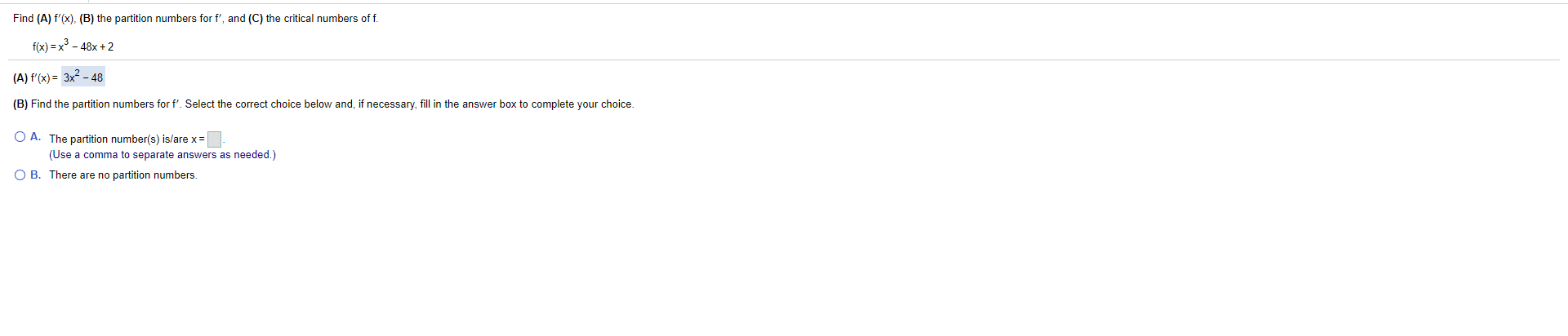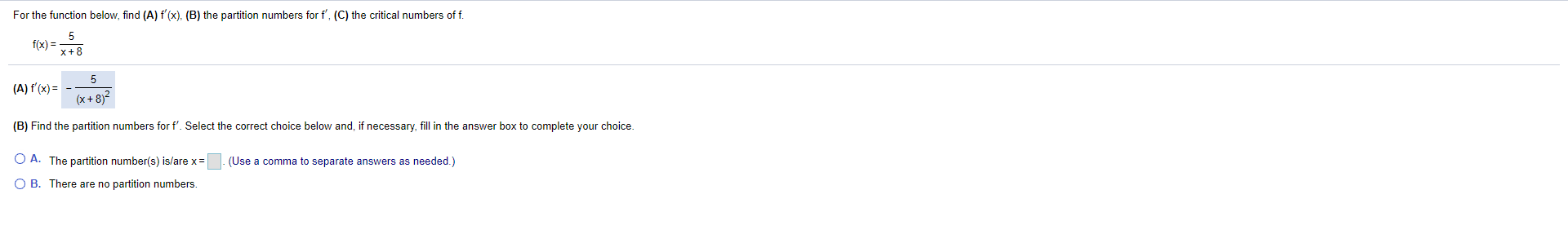### Create an Account

Already have account?

### Forgot Your Password ?

Home / Questions / Find (A) f'(x), (B) the partition numbers for f', and (C) the critical numbers of f. f(x) ...

# Find (A) f'(x), (B) the partition numbers for f', and (C) the critical numbers of f. f(x) = x3 - 48x+2 (A) f'(x)= 3x2 - 48 (B) Find the partition numbers for f'. Select the correct choice below and, i

Find (A) f'(x), (B) the partition numbers for f', and (C) the critical numbers of f. f(x) = x3 - 48x+2 (A) f'(x)= 3x2 - 48 (B) Find the partition numbers for f'. Select the correct choice below and, if necessary, fill in the answer box to complete your choice. O A. The partition number(s) is/are x = (Use a comma to separate answers as needed.) O B. There are no partition numbers.
For the function below, find (A) f'(x), (B) the partition numbers for f', (C) the critical numbers off. f(x) = 5 X + 8 (A) f'(x) = 5 (X + 8) 2 (B) Find the partition numbers for f'. Select the correct choice below and, if necessary, fill in the answer box to complete your choice. (Use a comma to separate answers as needed.) O A. The partition number(s) is/are x = OB. There are no partition numbers.Apr 14 2021 View more View Less

#### Answer (Solved)Subscribe To Get Solution